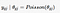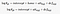# March Madness Predictions using PyMC3

## Presenting Two (Very Simple) Hierarchical Bayesian Models

This post describes my journey from exploring the model from Predicting March Madness Winners with Bayesian Statistics in PYMC3! by Barnes Analytics to developing a much simpler linear model.

(I struggled to define a target audience for this post. I don’t intend to make this post a tutorial for beginners. But experts will surely find it too simple. This post is in fact a form of soft documentation for myself, so it’ll be probably also be helpful to someone who is familiar of the concept of probabilistic programming and PyMC3 but needs some inspiration to get started.)

## PyMC3

Taken from the official documentation:

PyMC3 is a Python package for Bayesian statistical modeling and Probabilistic Machine Learning which focuses on advanced Markov chain Monte Carlo and variational fitting algorithms.

PyMC3 is Python-native, so I personally find it easier to use Stan. It is based on Theano, whose development has unfortunately stopped. The work to replace Theano seems to be ongoing. There have also been some new competitors closing in, e.g. Edward(based on Tensorflow) and Pyro(based on PyTorch).

# The First Model (Off. and Def. Score Decomposition)

As mentioned in the beginning of the post, this model is heavily based on the post by Barnes Analytics. The model seems to originate from the work of Baio and Blangiardo (in predicting footbal/soccer results), and implemented by Daniel Weitzenfeld. There is also an example in the official PyMC3 documentation that uses the same model to predict Rugby results.

The model decompose everything that influences the results of a game into five factors:

2. The offensive power of the home team
3. The defensive power of the home team
4. The offensive power of the away team
5. The defensive power of the away team

And it use a Possion regression (belongs to the generalized linear regression family) to model the relationship between these factors. It can be easily formalized by the following formulae from Daniel Weitzenfeld’s post:(Theta is the “event rate” of a Poisson distribution)The log of a theta is calculated from a linear combination of factors

I’m not going to spend time explaining how to do data preprocessing here. Please check the Barnes Analytics post or this Kaggle Kernel. I’ve made some changes to the Barnes Analytics code. Besides some small code tweaks, there are two bigger changes:

1. Expand the PyMC model to fit multiple seasons at once
2. Vectorize the simulation function used to calculate the winning/losing probabilities.

## Fit Multiple Seasons

Originally the model can only fit data from only one season (in the following case, the 2017 season):

`model = pymc3.Model()with model:    # global model parameters    home = pymc3.Flat('home')    sd_att = pymc3.HalfStudentT('sd_att', nu=3, sd=2.5)    sd_def = pymc3.HalfStudentT('sd_def', nu=3, sd=2.5)    intercept = pymc3.Flat('intercept')        # team-specific model parameters    offs_star = pymc3.Normal(        'offs_star', mu=0, sd=sd_att, shape=num_teams)    defs_star = pymc3.Normal(        'defs_star', mu=0, sd=sd_def, shape=num_teams)    # sum-to-zero    offs = pymc3.Deterministic(        'offs', offs_star - tt.mean(offs_star))    defs = pymc3.Deterministic(        'defs', defs_star - tt.mean(defs_star))        # derive the scoring intensity for a game    home_theta = tt.exp(        intercept + home * advantage +           offs[df_regular_2017["HomeTeamID"].values] +         defs[df_regular_2017["AwayTeamID"].values])    away_theta = tt.exp(        intercept  +         offs[df_regular_2017["AwayTeamID"].values +         defs[df_regular_2017["HomeTeamID"].values])        # likelihood of observed data    home_points = pymc3.Poisson(        'home_points', mu=home_theta,              observed=df_regular_2017["HomeScore"].values)    away_points = pymc3.Poisson(        'away_points', mu=away_theta,         observed=df_regular_2017["AwayScore"].values)`

(The operations starts with “tt.” are Theano tensor operations. ) Note the model implements a sum-to-zero constraint by subtracting the global mean. Otherwise we can shift all offensive power by z , all defensive power by -z and still get the same model.

The simplest way to fit more than one season in one run is to assign separate offensive and defensive powers to each team for each season, and assume there are no correlations between seasons (You can also simply loop through each season and fit one model per season under this assumption.):

`model = pymc3.Model()with model:    # global model parameters    home = pymc3.Flat('home')    sd_att = pymc3.HalfStudentT('sd_att', nu=3, sd=2.5)    sd_def = pymc3.HalfStudentT('sd_def', nu=3, sd=2.5)    intercept = pymc3.Flat('intercept')        # team-specific model parameters    offs_star = pymc3.Normal(        'offs_star', mu=0, sd=sd_att, shape=(5, num_teams))    defs_star = pymc3.Normal(        'defs_star', mu=0, sd=sd_def, shape=(5, num_teams))    offs = pymc3.Deterministic(        'offs', offs_star - tt.mean(offs_star))    defs = pymc3.Deterministic(        'defs', defs_star - tt.mean(defs_star))        # derive the scoring intensity for a game    home_theta = tt.exp(        intercept + home * advantage +         offs[df_regular["SeasonID"].values,             df_regular["HomeTeamID"].values] +         defs[df_regular["SeasonID"].values,             df_regular["AwayTeamID"].values])    away_theta = tt.exp(        intercept +         offs[df_regular["SeasonID"].values,              df_regular["AwayTeamID"].values] +         defs[df_regular["SeasonID"].values,              df_regular["HomeTeamID"].values])        # likelihood of observed data    home_points = pymc3.Poisson(        'home_points', mu=home_theta,         observed=df_regular["HomeScore"].values)    away_points = pymc3.Poisson(        'away_points', mu=away_theta,         observed=df_regular["AwayScore"].values)`

(The above example uses Season 2014 to 2018. SeasonID = Season-2014)

If we want to model the correlations between seasons, one way to do it is to base the score/power of one team in this season on the last season. For example, we can make a team’s score in season 2 the sum of its score in season 1 and a white noise. There’s a GaussianRandomWalk class in PyMC3 that does exactly this. However, I somehow could not make GaussianRandomWalk to work with multiple series (teams). So instead I manually defined scores season by season and linked them together. The results from this model are not better than assuming independence between seasons, so I’ll skip the code for this one.

## Faster Simulation

The original simulation function to generate winning probabilities:

`def calculate_winning_probability(        trace, season=0, team_1=0, team_2=1, sample_size=100):    wins = []    for i in range(sample_size):        draw = np.random.randint(0, 1000)        intercept_ = trace['intercept'][draw]        offs_ = trace['offs'][draw]        defs_ = trace['defs'][draw]        home_theta_ = np.exp(            intercept_ + offs_[season, team_1] +             defs_[season, team_2])        away_theta_ = np.exp(            intercept_ + offs_[season, team_2] +             defs_[season, team_1])        home_score_ = np.random.poisson(home_theta_, 1)        away_score_ = np.random.poisson(away_theta_, 1)        wins.append((home_score_ - away_score_ > 0).astype("int"))    return np.mean(wins)`

The code inside the loop takes a set of samples from the posterior distributions, calculates the corresponding thetas, sample from the resulting Poisson distributions, and see if the home team has a higher score than the away team. The code is repeated sample_size times and the winning ratio of the home team is our predicted winning probability.

The code can be vectorized to utilize the optimized code of Numpy and Theano:

`def calculate_winning_probability(        trace, season=0, team_1=0, team_2=1, sample_size=100):    draw = np.random.randint(        0, trace['intercept'].shape, size=sample_size)    intercept_ = trace['intercept'][draw]    offs_ = trace['offs'][draw]    defs_ = trace['defs'][draw]    home_theta_ = np.exp(        intercept_ + offs_[:, season,  team_1] +         defs_[:, season,  team_2])    away_theta_ = np.exp(        intercept_ + offs_[:, season,  team_2] +         defs_[:, season,  team_1])    home_score_ = np.random.poisson(        home_theta_, sample_size)    away_score_ = np.random.poisson(        away_theta_, sample_size)       wins = np.mean((home_score_ - away_score_ > 0))    return (        wins,        (np.percentile(home_score_, 5),          np.percentile(home_score_, 50),         np.percentile(home_score_, 95)),         (np.percentile(away_score_, 5),          np.percentile(away_score_, 50),         np.percentile(away_score_, 95))    )`

The vectorized function also returns the 5, 50, and 95 percentiles of the simulated home_score and away_score for later model diagnostics.

The speedup (tested on a single season model, but should make no difference anyway):

In fact, if we take the percentile calculation away, the vectorized function can achieve a 1000x speedup (~2.6ms) instead of a 100x speedup.

## Model Evaluation

Take this Kaggle kernel/notebook as an example. The model was fitted to the results of regular season matches:

(The variance of the offensive and defensive power distribution seems to become smaller when the mean of distribution becomes larger. I’m not sure why.)

Now we use the tournaments in March to test our predictions:

(Correction: it’s really a 90% confidence interval instead of 95% in the screenshot) Only around 85% of the time the actual score fell into the 90% confidence interval, so the model is far from ideal.

As for accuracy, overall accuracy is 67.54% (the percentage where the model predicted the winner correctly), and for 2017 season the accuracy is 67.16%. The overall and 2017 logloss is 0.5388 and 0.5654, respectively.

# The Second Model (Single Score per Team)

One of my favorite things to do is to take a problem and try to find the simplest model that can solve the problem to a reasonable degree. For this prediction problem, I want to assign only one score per team, use the difference of the scores between two teams to predict the difference of their final points in a game. So there are down to only three factors influencing the results of a match:

2. The score of the home team
3. The score of the away team

(I’m using points specifically for the points made in matches, and scores for the latent variables we created in this section to avoid confusion.)

The relationship between the difference of the final points and the factors is entirely linear, i.e. `point_difference = home_advantage + score_team_1 — score_team_2`. I know, it seems to incredibly over-simplify things, but does it?

## The model

`model = pymc3.Model()with model:    # global model parameters    home = pymc3.Flat('home')    sd_scores = pymc3.HalfStudentT('sd_scores', nu=10, sd=10)    sd_points_diff = pymc3.HalfStudentT('sd_diff', nu=20, sd=10)    # team-specific model parameters    scores_ = pymc3.Normal(        "scores_raw", mu=0, sd=sd_scores, shape=(5, num_teams))    scores = pymc3.Deterministic(        "scores", scores_ - tt.mean(scores_, axis=0))        points_diff = pymc3.Normal('points_diff',         home * advantage +         scores[df_regular["SeasonID"].values,                df_regular["HomeTeamID"].values] -         scores[df_regular["SeasonID"].values,                df_regular["AwayTeamID"].values],        sd=sd_points_diff,        observed=df_regular["ScoreDiff"].values)`

(Although the differences of the final score are discrete, using a Gaussian distribution to approximate it should not be a big problem) Extremely simple code. There are two random variables set as the standard deviations, the (latent) variables for (season, team), and one random variable for the home court advantage.

## Model Evaluation

Take this Kaggle kernel/notebook as an example:

Because this is a linear model, the results are very interpretable. Home team get an advantage of around 3.4 points in average, which is not a lot, but already requires two field goals to counter. The standard deviation of team scores is around 8.6, and the one of point differences is around 10.35. They are both quite large, which means the uncertainty of the predictions are high.

(Correction: it’s really a 90% confidence interval instead of 95% in the screenshot) 91.79% of the actual point differences fell into the 90% confidence interval. It seems acceptable, and is far better than the first model. (Most intervals are pretty wide, though.)

As for accuracy, overall accuracy is 69.78% (the percentage where the model predicted the winner correctly), and for 2017 season the accuracy is 70.15%. The overall and 2017 logloss is 0.5007 and 0.5349, respectively.

# Concluding Remarks

Surprisingly, the second model performs better than the first model. In additional to the 2%+ improvement in accuracy, the better logloss values also indicate a better quality in probability predictions.

Admittedly, 70% accuracy is still nothing to be proud of, but we can build more sophisticated models based on it by adding more features or data. For example, we can add an adjustment term to account for the fact that some teams play better against better teams, while only mediocre against equal or worse teams. It’s also common sense that basket ball cannot be truly linear. Even if team A beats team B by 10 points and team B beats team C by 10 points, team A beating team C by 20 points is definitely not a sure thing (It’d probably often be less than that). How to better model that dynamics is for you to find out.

## Source Code (as Kaggle Kernels)

The first model (offensive and defensive decomposition):

1. Single Season
2. Multiple Seasons (Global sum-to-zero)

The second model (single score per team):

1. Multiple Seasons

## 20180403 Update — Visualization

This visualization tool written by Mark McClure is really good. The quality of your prediction is shown in colors, and hovering over a match will show the probability you predicted:

You have to follow Kaggle’s submission format, though. Check it here and here.

Written by

Written by

## Ceshine Lee

#### Humanist. Data Geek. Twitter: @ceshine_en## More From Medium

Welcome to a place where words matter. On Medium, smart voices and original ideas take center stage - with no ads in sight. Watch
Follow all the topics you care about, and we’ll deliver the best stories for you to your homepage and inbox. Explore
Get unlimited access to the best stories on Medium — and support writers while you’re at it. Just \$5/month. Upgrade